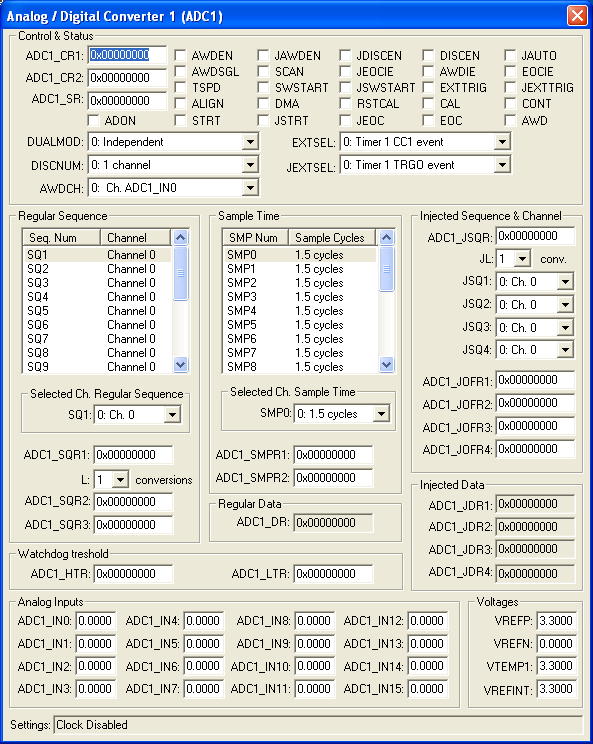Products Download Events Support Videos All Product Families ARM7, ARM9, and Cortex-M3 Products C16x, XC16x, and ST10 Products C251 and 80C251 Products Cx51 and 8051 Products Modified Anytime In the Last 7 Days In the Last 14 Days In the Last 30 Days In the Last 90 Days In the Last 6 Months

# Peripheral Simulation

For STMicroelectronics STM32F103C8 — Analog/Digital Converter 1 (ADC1)

Simulation support for this peripheral or feature is comprised of:

• Dialog boxes which display and allow you to change peripheral configuration.
• VTREGs (Virtual Target Registers) which support I/O with the peripheral.

These simulation capabilities are described below.

### Analog / Digital Converter DialogThe Analog / Digital Converter dialog displays and configures the A/D converter. The controls in this dialog are separated into several logical groups.

Control and Status

• ADC_CSR  (ADC Control & Status Register) contains the following control and status settings:
• A (Channel Address) selects the channel to be sampled when external addressing (AXT) is enabled.
• OR (Overrun) is set when one of the data channels is overwritten before being read.
• AXT (Addressing External Enable) is set to allow single-channel conversion. If reset, channels are addressed in a round-robin fashion.
• IEn (Interrupt Enable - Channel n) is set to enable interrupts for the corresponding channel n.
• DAn (Data Available - Channel n) is set when new sample data is ready to be read for channel n.

Prescaler

• ADC_CPR (ADC Prescaler Register) contains the following prescaler settings:
• PRESC (Prescaler Value) is the 12-bit PCLK2 clock divisor used to produce the oversampling clock.
• Fmod(Hz) (Modulator Oversampling Frequency) is the sampling frequency input to the ADC.
• Rate(Hz) (Modulator Oversampling Rate) is oversampling rate based on the prescaling factor (PRESC).

Data

• ADC_DATAn (ADC Data Register) contains the raw converted result of the ADC conversion for channel n.
• DATAn (Converted Data) contains the converted data value for channel n.

• AINn (Analog Input) contains the simulated analog input voltage (VTREG AINn) for channel n.

Reference & Center

• VREF is the feedback reference voltage used for the ADC.
• VCM is the midpoint between the voltage range of the ADC converter and the feedback reference voltage (VREF).

### ADC1_INx Input Voltage VTREGData Type: float

The ADC1_INx VTREGs set the analog input voltages for simulated A/D converters. The ADC1_INx VTREGs represent the inputs to the analog input pins of the MCU for analog input 0, 1, 2, and so on. ADC1_IN0 sets the input voltage for analog input #0, ADC1_IN1 sets the input voltage for analog input #1, etc. If you have properly configured the analog inputs, the following commands (typed in the debugger's Command Window) input the voltages specified.

```ADC1_IN0 = 0.000  /* Analog Input 0 = 0.000 volts */
ADC1_IN1 = 2.500  /* Analog Input 1 = 2.500 volts */
ADC1_IN2 = 4.999  /* Analog Input 2 = 4.999 volts */
```

You may create a debugger signal function to periodically change the value of the A/D input. The following signal function increases the A/D Channel 1 input voltage by 0.1 volts each second.

```signal void ADC1_IN1_sig (void) {
float f;

for (f = 0.0; f < 5.0; f += 0.1)
{
swatch (1.0);                  // Delay 1 second
ADC1_IN1 = f;                  // Set ADC1_INx1 Voltage
}
}
```